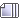###Author Topic: The one unforgivable sin is to be boring  (Read 715 times)

0 Members and 0 Guests are viewing this topic.

#### mic check##### Infinite series approximating decimal fractions
« Reply #15 on: February 01, 2020, 05:36:52 pm »
Given 0.abcde.... ===> (implies) 0 + a/10 + b/100 + c/1000 + ...

The Limit as the number of decimal fraction elements/components of the Sum increases toward infinity, with each element/component becoming smaller by 1/10 can be represented as a decimal fraction a/b where a,b are Integers and b != 0.

example:  0.222 where 2 would have a bar over it ....

[to be continued after I cook The Mother's dinner].0.222  = 0 + 0.2 + 0.02 + 0.002 + 0.0002 + ...

This is a geometric progression with a = 0.2 and r = 0.1

Hence, the limit as n approaches infinity is SUM = 0.2/(1-0.1) = 0.2/0.9 = 2/9

I will find some pages about the observations on the binomial expansion (x + y)^n then will upload.

We each have different "down times" -----
« Last Edit: February 01, 2020, 06:11:11 pm by mwh »
Things They Will Never Tell YouArthur Schopenhauer has been the most radical and defiant of all troublemakers.

Gorticide @ Nothing that is so, is so DOT edu

~ Tabak und Kaffee Süchtigen ~